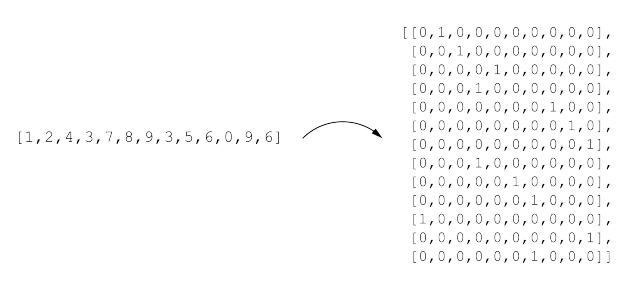# Model not learning at all using pretrained Resnet50

Hello everyone, my team and I are trying to develop a model capable of reading EAN13 barcodes using a camera.

On that purpose we have developed a model using Resnet50 pretrained model in PyTorch, adapting it to have an output of 130 classes (each digit in the code is represented by a one hot encoding of 10 numbers).The problem is that the model is not converging at all. Loss is stuck at a value of 29 and accuracy is around 0.10.

Does anyone have an idea of what we can change to make the net actually learn? Thank you very much in advance for any advice.

My net is represented like this right now:

``````from torch import nn, optim
import torch.nn.functional as F
from torchvision import models
from torchvision.models.resnet import ResNet50_Weights

# Define ResNet50 model
class ResNet50(nn.Module):
def __init__(self, num_classes):
super(ResNet50, self).__init__()
self.resnet50 = models.resnet50(weights=ResNet50_Weights.DEFAULT)
self.fc1 = nn.Linear(1000, 256)
self.fc2 = nn.Linear(256, num_classes)

def forward(self, x):
x = self.resnet50(x)
x = x.view(x.size(0), -1)
x = self.fc1(x)
x = F.relu(x)
x = self.fc2(x)
return x
``````

And my training code is as follows:

``````import torch
from EAN13Dataset import EAN13Dataset
from torch import nn, optim
from tqdm import tqdm
from resnet50_model import ResNet50EAN13
import datetime
import glob

import gc

torch.cuda.empty_cache()
gc.collect()

BATCH_SIZE = 64

train_dataset = EAN13Dataset("dataset", split="train")

valid_dataset = EAN13Dataset("dataset", split="valid")

device = torch.device("cuda" if torch.cuda.is_available() else "cpu")

# Create model instance
model = ResNet50EAN13(num_classes=13 * 10)  # 13 digits with 10 possible values each
model = model.to(device)
model.train()

# Define loss function and optimizer
criterion = nn.CrossEntropyLoss()

train_dataset = glob.glob('dataset/train/*/*.jpg')
print(f'Conjunto de entrenamiento: {len(train_dataset)} imágenes')

valid_dataset = glob.glob('dataset/valid/*/*.jpg')
print(f'Conjunto de validación: {len(valid_dataset)} imágenes')

# Train the model
num_epochs = 20

for epoch in range(num_epochs):
# Train
for _, inputs, *labels in progression_bar:
inputs = inputs.to(device)
labels = [l.to(device) for l in labels]

outputs = model(inputs)
losses = []
for i in range(len(labels)):
loss = criterion(outputs[:, i * 10 : (i + 1) * 10], labels[i])
losses.append(loss)
loss = sum(losses)
progression_bar.set_description(f"Epoch {epoch+1} -- CrossEntropyLoss: {loss}")
loss.backward()
optimizer.step()

# Evaluate
accuracy = 0.0
for _, inputs, *labels in valid_loader:
inputs = inputs.to(device)
labels = [l.to(device) for l in labels]

outputs = model(inputs)
num_correct = 0
for i in range(len(labels)):
_, predicted = torch.max(outputs[:, i * 10 : (i + 1) * 10], dim=1)
labels_decoded = torch.argmax(labels[i], dim=1)
num_correct += torch.sum(predicted == labels_decoded)
accuracy += num_correct / (len(labels) * inputs.size(0))
I’m unsure if I understand your use case correctly but it seems your model is supposed to output one-hot encoded logits which you are later slicing? Note that `nn.CrossEntropyLoss` is used for a multi-class classification use case where each sample belongs to one class only. Is this the reason you are slicing the output to “fake” multiple output heads?